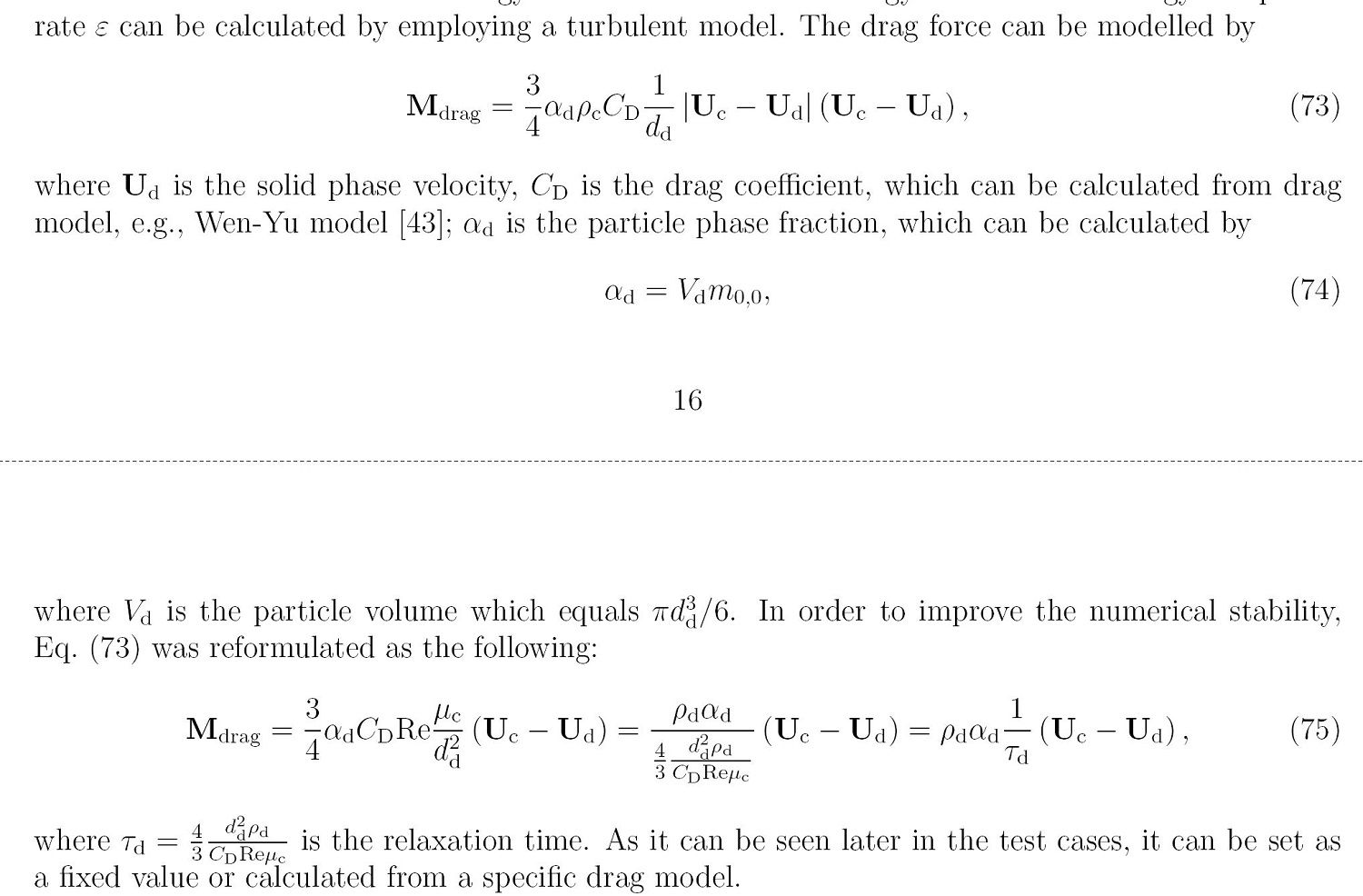# drag force in dragModel.C

• Hi, everyone. I am confused that the expression of drag force used in Openfoam, as shown in the following codes. Why there is nu and divided by square of particle diameter. I saw the formula is without nu and only divided by diameter in some literature. Do you have any tip for me? Thanks a lot. By the way, After I deleted pair_.continuous().nu() and only divided by diameter. The results I obtained are totally the same.

Foam::tmp<Foam::volScalarField> Foam::dragModel::Ki() const
{
return
0.75
*CdRe()
*swarmCorrection_->Cs()
*pair_.continuous().rho()
*pair_.continuous().nu()
/sqr(pair_.dispersed().d());
}


• 75最前面• Thanks a lot, Dongyue. Very helpful. I found in SN model CdRe was multiplied by another Re. Thus, the formula is consistent.

CFD中文网 2016 - 2020 | 京ICP备15017992号-2